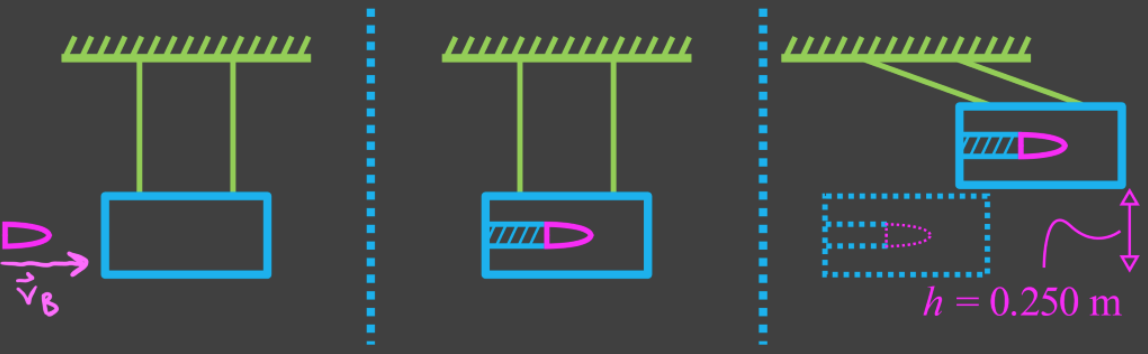# Elastic and inelastic collisions#### Everything You Need in One Place

Homework problems? Exam preparation? Trying to grasp a concept or just brushing up the basics? Our extensive help & practice library have got you covered.#### Learn and Practice With Ease

Our proven video lessons ease you through problems quickly, and you get tonnes of friendly practice on questions that trip students up on tests and finals.#### Instant and Unlimited Help

Our personalized learning platform enables you to instantly find the exact walkthrough to your specific type of question. Activate unlimited help now!##### Intros
###### Lessons
1. Introduction to elastic and inelastic collisions
2. Meaning of elastic and inelastic collisions
3. What happens to kinetic energy in a collision?
4. Understanding perfectly inelastic collisions
##### Examples
###### Lessons
1. Solving word problems with momentum and elastic/inelastic collisions
1. Two cars collide head-on and stick together. The cars are stationary after colliding.
1. Is total momentum conserved?
2. Is total energy conserved?
3. Is the collision elastic or inelastic?

2. Identify each situation as an inelastic or elastic collision.
1. One car crashes into another, bouncing apart with a loud bang.
2. A hammer strikes a piece of steel, bouncing off and producing sparks.
3. A He atom collides with a H atom, bouncing off and maintaining overall kinetic energy.

2. $\bold{\sum\vec{E}_i = \sum\vec{E}_f}$ $\bold{ ; }$ $\bold{\sum\vec{p}_i = \sum\vec{p}_f:}$ Conservation of energy and momentum in elastic and inelastic collisions
1. 3.6×104 kg train car A travelling at 5.40 m/s [E] collides with stationary 5.20×104 kg train car B. The train cars bounce apart, and after the collision, train car A travels at 1.70 m/s [E]. Determine if this collision is elastic or inelastic.
2. 0.50 kg steel ball A travelling [E] with a kinetic energy of 0.49 J collides with stationary 0.75 kg steel ball B head-on. After the collision, ball A travels at 0.28 m/s [W]. Assuming the collision is elastic, find the velocity of ball B after the collision.
3. A "ballistic pendulum" is a method used to measure the velocity of a bullet. A 5.60 g bullet is fired at a 1.24 kg wooden block suspended as shown in the diagram, and the block rises to 0.250 m higher than its initial position at the peak of its swing.
1. Find the velocity of the bullet when it hits the block.
2. Calculate how much of the kinetic energy is lost in this inelastic collision. Explain what happens to this energy.###### Topic Notes

In this lesson, we will learn:

• Meaning of elastic and inelastic collisions
• What happens to kinetic energy in a collision?
• Understanding perfectly inelastic collisions
• Problem solving with elastic and inelastic collisions

Notes:

• Total momentum and total energy are conserved in collisions. However, kinetic energy is not always conserved, since it can be converted into other forms of energy.
• Elastic collision: collision where no kinetic energy is lost
• Inelastic collision: collision where part of the kinetic energy is converted to other forms of energy
• Perfectly inelastic collision: collision where the maximum possible amount of kinetic energy is converted to other forms of energy; objects stick together.

Conservation of Momentum

$\sum\vec{p}_i = \sum\vec{p}_f$

$\vec{p}_i:$ initial momentum, in kilogram meters per second (kg·m/s)

$\vec{p}_f:$ final momentum, in kilogram meters per second (kg·m/s)

Conservation of Energy

$\sum\vec{E}_i = \sum\vec{E}_f$

$\vec{E}_i:$ initial energy, in joules (J)

$\vec{E}_f:$ final energy, in joules (J)

Kinetic Energy

$KE = \frac{1}{2}mv^2$

$KE:$ kinetic energy, in joules (J)

$m:$ mass, in kilograms (kg)

$v:$ speed, in meters per second (m/s)

Potential Energy

$PE = mgh$

$PE:$ potential energy, in joules (J)

$g:$ acceleration due to gravity, in meters per second squared (m/s2)

$h:$ height, in meters (m)